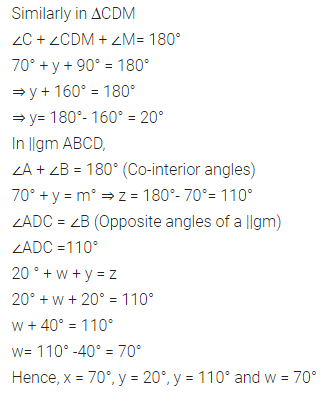# ML Aggarwal Class 8 Solutions for ICSE Maths Model Question Paper 4

## ML Aggarwal Class 8 Solutions for ICSE Maths Model Question Paper 4

Choose the correct answer from the given four options (1-2):
Question 1.
$$\frac{103^{2}-97^{2}}{200}$$ is equal to
(a) 3
(b) 4
(c) 5
(d) 6
Solution: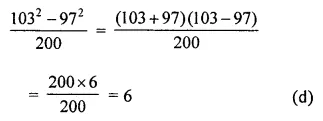Question 2.
If the’ sum of three consecutive even integers is 36, then the largest integer is
(a) 10
(b) 12
(c) 14
(d) 16
Solution:Question 3.
Find the area of rectangle whose length and breadth are respectively (4x2 – 3x + 7) and (3 – 2x + 3x2).
Solution: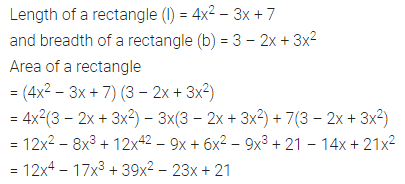Question 4.
Factorize: a2 – c2 – 2ab + b2.
Solution: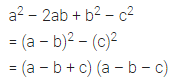Question 5.
The ages of A and B are in the ratio 3 : 4. Five years later the sum of their ages will be 31 years. What are their present ages?
Solution: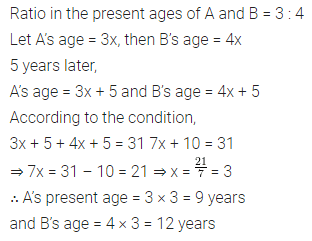Question 6.
The sum of the digits of a two-digit number is 13. If the number obtained by reversing the digits is 45 more than the original number. Find the original number.
Solution:Question 7.
The ratio between an exterior angle and interior angle of a regular polygon is 1 : 5, find:
(i) the measure of each exterior angle,
(ii) the measure of each interior angle.
(iii) the number of sides of the polygon.
Solution: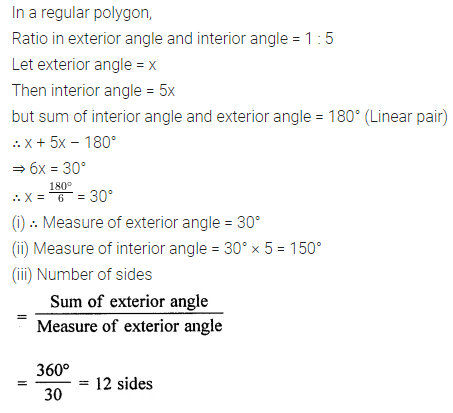Question 8.
Solve the inequality: 3 – $$\frac{x}{2}$$ > 2 – $$\frac{x}{3}$$ , x ϵ W.
Also represent its solution set on the number line.
Solution:Question 9.
Factorise: x2 + $$\frac{1}{x^{2}}-7\left(x-\frac{1}{x}\right)$$ + 8.
Solution: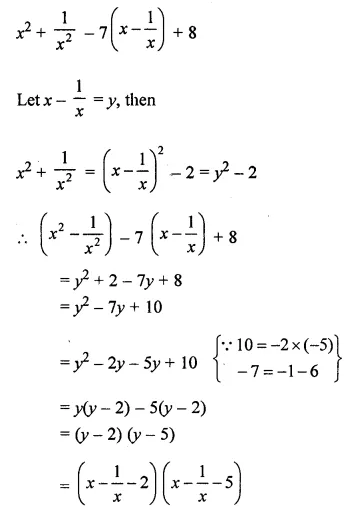Question 10.
In the given figure, ABCD is a parallelogram. Find x, y, z and w.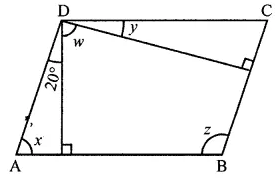Solution: